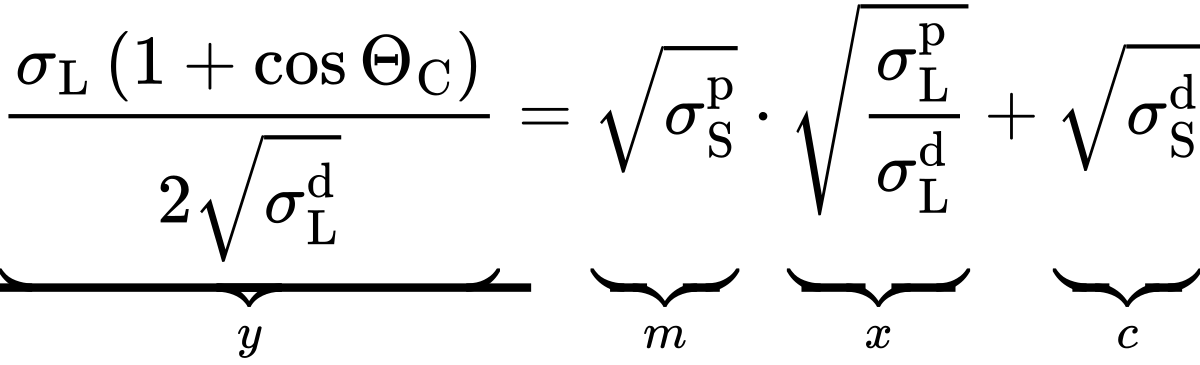Surface energy of a solid# Determination of the surface energy of a solid

To determine the surface energy of a solid one measures the contact angles of test liquids whose surface tensions including their dispersive and polar parts are known. These dispersive and polar parts are used to calculate the interfacial tension between the solid and a liquid based on a suitable model. An often applied model is the one of Owens, Wendt, Rabel and Kaelble (OWRK-model) which considers the geometric mean of the dispersive and polar parts of the liquid's surface tension and of the solid's surface energy:Substituting this expression in the Young equation a linear equation of the type y=mx+c can be obtained:Figure 1: Regression line for determination of surface energy

In this equation y and x contain the known quantities, namely the measured contact angle and the dispersive and polar parts of the test liquid's surface tension. The searched dispersive and polar parts of the solid's surface energy are contained in the axis intercept c and in the slope m, respectively. These parameters can be evaluated creating a regression line (see figure 1) when contact angles of at least two test liquids are measured.

However, as a regression line based on just two points contains no information on the accuracy of the result, contact angle measurements with at least three test liquids are recommended. Generally, the use of diiodomethane, ethylene glycol and thiodiglycol as test liquids yields good results. The OCA software performs the described evaluation automatically and includes, in addition to the OWRK-model, eight more theories to determine the surface energy of a solid.

# Chapters

Using the following buttons you can navigate between the individual chapters of our knowledge-base Understanding Interfaces